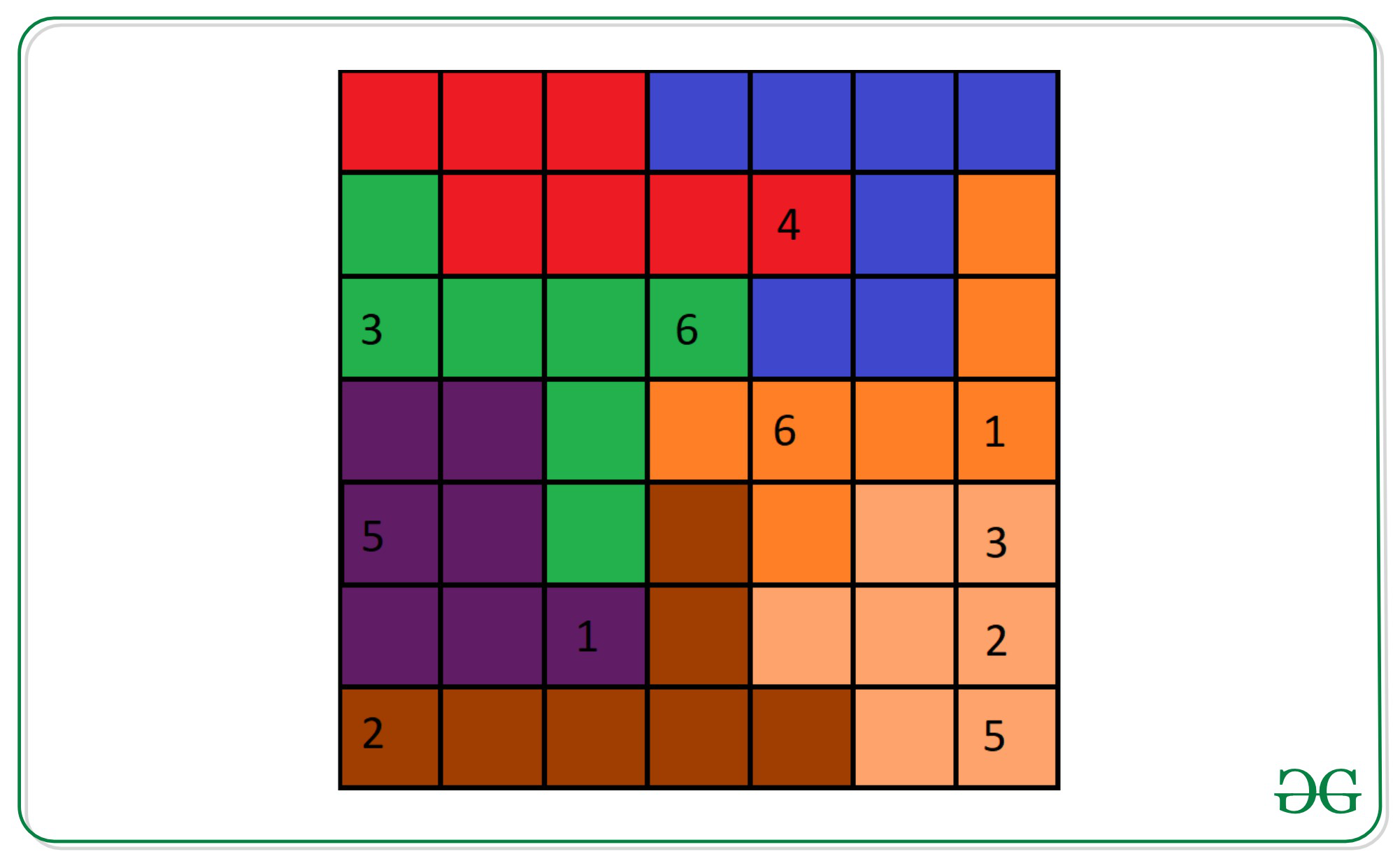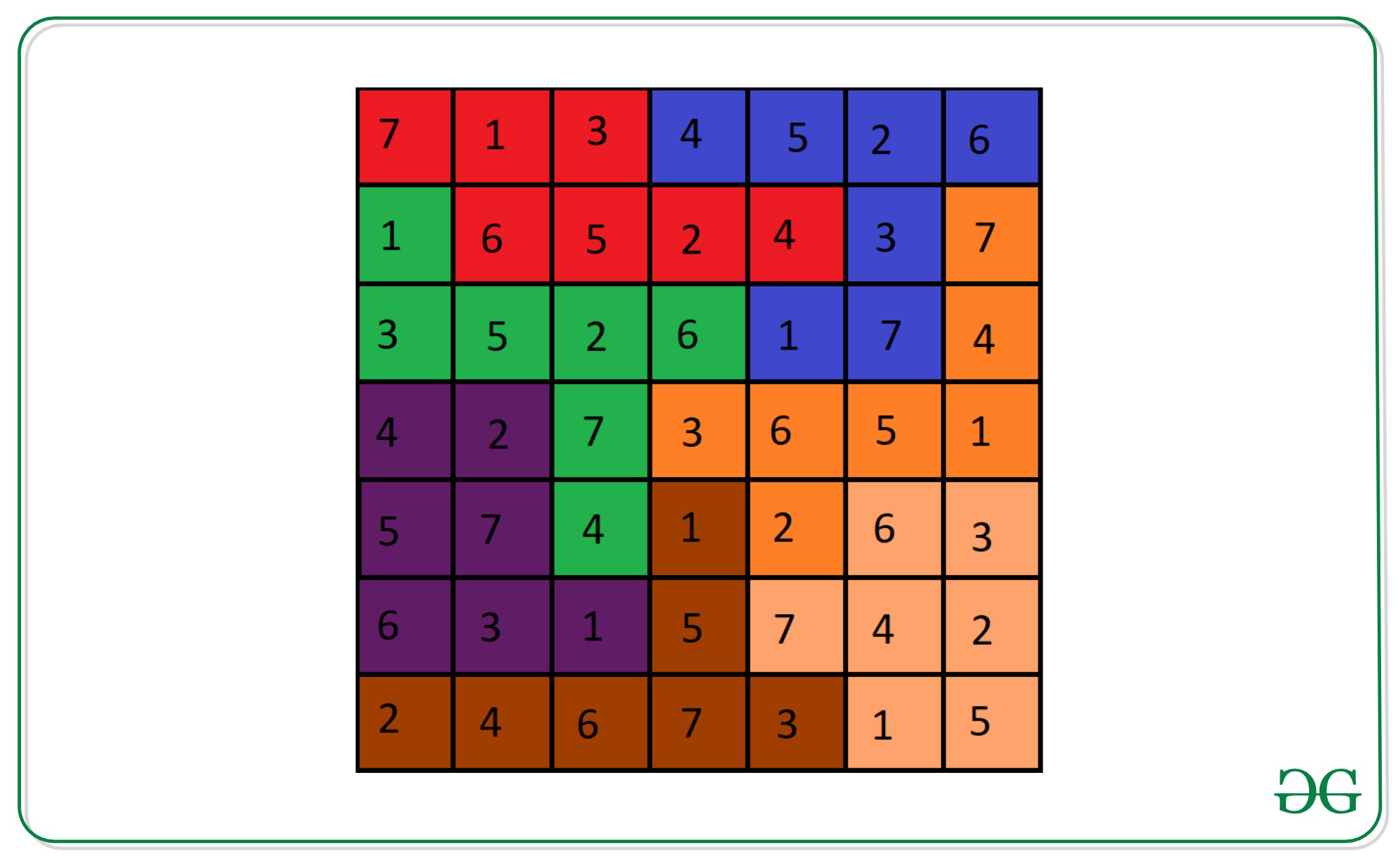# Solve Sudoku on the basis of the given irregular regions

• Last Updated : 09 May, 2022

Given two matrices sudoku[][] and region[][] of size N * N, the task is to fill the given Sudoku on the basis of the given irregular regions. If it is not possible to fill the sudoku[][] matrix, then print -1. Following are the definitions of the matrices:

Sudoku Matrix (sudoku[][]): It is an N×N matrix that contains the elements from 0 to N, where 0 denotes the empty cell which needs to be filled in order to solve the Sudoku.
Rules to solve the Sudoku:

• Each Row and column must have unique numbers from 1 to N.
• Each region must have unique numbers from 1 to N.

Region Matrix (region[][]): It is an N×N matrix containing characters which denote the region of the Sudoku. Same characters in matrix denote a region of Sudoku.

Examples:

Input: sudoku[][] = {
{0, 0, 0, 0, 0, 0, 0},
{0, 0, 0, 0, 4, 0, 0},
{3, 0, 0, 6, 0, 0, 0},
{0, 0, 0, 0, 6, 0, 1},
{5, 0, 0, 0, 0, 0, 3},
{0, 0, 1, 0, 0, 0, 2},
{2, 0, 0, 0, 0, 0, 5} }
region[][] = {
{r, r, r, b, b, b, b},
{g, r, r, r, r, b, o},
{g, g, g, g, b, b, o},
{p, p, g, o, o, o, o},
{p, p, g, d, o, l, l},
{p, p, p, d, l, l, l},
{d, d, d, d, d, l, l} }
Output:
7 1 3 4 5 2 6
1 6 5 2 4 3 7
3 5 2 6 1 7 4
4 2 7 3 6 5 1
5 7 4 1 2 6 3
6 3 1 5 7 4 2
2 4 6 7 3 1 5
Explanation:
Unsolved Sudoku Puzzle with the regions:Solved Sudoku puzzle with the regions:Input: sudoku[][] = {
{ 0, 0 },
{ 0, 1 } },
region[][] = {
{r, r},
{b, b}}
Output:
1 2
2 1
Explanation:
Elements of the row of the output matrix: {1, 2}, {2, 1}
Elements of the column of the matrix: {1, 2}, {2, 1}
Elements of the same regions of the matrix: {1, 2}, {2, 1}
Since it contains unique elements in the rows, columns and regions.
Therefore, it is a valid solution of the Sudoku.

Approach: The idea is to use Backtracking to solve the Sudoku by assigning the number to empty cells one by one recursively and backtrack if any of the rules are violated i.e., each row, column, and region must have unique numbers from 1 to N. Below is the illustration with the help of steps:

• Create a function to check if the assigned number to a cell is safe or not:
• For checking in the current row and column, iterate over the row and column and check if the assigned number is present in the cell or not.
• For checking whether the number is present in the current region or not, use Breadth-First Search.
• Iterate over the grid to check for any unassigned cell and assign the values from 1 to N and check if the number assigned is safe or not. If the number assigned is not safe then back-track for the unassigned cell.
• If all the unassigned cells are assigned by some number, then print the Sudoku. Otherwise, there is no solution possible.

Below is the implementation of the above approach:

## C++

 `// C++ program for the above approach` `#include ``using` `namespace` `std;` `// Grid dimension``const` `int` `N = 2;` `// Function to check if the number to be present``// in the current cell is safe or not``bool` `issafe(``int` `sudoku[N][N], ``int` `i, ``int` `j, ``int` `n,``            ``int` `number, ``char` `region[N][N])``{` `    ``// Check if the number is present in``    ``// i-th row or j-th column or not``    ``for` `(``int` `x = 0; x < n; x++) {``        ``if` `(sudoku[x][j] == number``            ``|| sudoku[i][x] == number) {``            ``return` `false``;``        ``}``    ``}` `    ``// Check if the number to be filled``    ``// is safe in current region or not``    ``char` `r = region[i][j];` `    ``// Initialize the queue for the BFS``    ``queue > q;` `    ``// Insert the current cell into queue``    ``q.push(make_pair(i, j));` `    ``// Check if the neighbours cell is``    ``// visited or not``    ``int` `visited[N][N];` `    ``// Initialize visited to 0``    ``memset``(visited, 0, ``sizeof` `visited);` `    ``// Mark current cell is visited``    ``visited[i][j] = 1;` `    ``// Performing the BFS technique``    ``// Checking for 4 neighbours at a time``    ``while` `(!q.empty()) {` `        ``// Stores front element of the queue``        ``pair<``int``, ``int``> front = q.front();` `        ``// Pop top element of the queue``        ``q.pop();` `        ``// Check for neighbours cell``        ``if` `(front.first + 1 < N``            ``&& region[front.first + 1][front.second] == r``            ``&& !visited[front.first + 1][front.second]) {` `            ``// If already contains the same number``            ``if` `(sudoku[front.first + 1][front.second]``                ``== number) {``                ``return` `false``;``            ``}``            ``q.push(make_pair(front.first + 1,``                             ``front.second));` `            ``// Mark as neighbour cell as visited``            ``visited[front.first + 1][front.second] = 1;``        ``}` `        ``// Checking for 2nd neighbours``        ``if` `(front.first - 1 >= 0``            ``&& region[front.first - 1][front.second] == r``            ``&& !visited[front.first - 1][front.second]) {` `            ``// If neighbours contains the same number``            ``if` `(sudoku[front.first - 1][front.second]``                ``== number) {``                ``return` `false``;``            ``}` `            ``// Insert neighbour cell into queue``            ``q.push(make_pair(front.first - 1,``                             ``front.second));` `            ``// Mark neighbour cell as visited``            ``visited[front.first - 1][front.second] = 1;``        ``}` `        ``// Checking for 3rd neighbours``        ``if` `(front.second + 1 < N``            ``&& region[front.first][front.second + 1] == r``            ``&& !visited[front.first][front.second + 1]) {` `            ``// If neighbours contains the same number``            ``if` `(sudoku[front.first][front.second + 1]``                ``== number) {``                ``return` `false``;``            ``}` `            ``// Insert neighbour cell into queue``            ``q.push(make_pair(front.first,``                             ``front.second + 1));` `            ``// Mark neighbour cell as visited``            ``visited[front.first][front.second + 1] = 1;``        ``}` `        ``// Checking for 4th neighbours``        ``if` `(front.second - 1 >= 0``            ``&& region[front.first][front.second - 1] == r``            ``&& !visited[front.first][front.second - 1]) {` `            ``// If neighbours contains the same number``            ``if` `(sudoku[front.first][front.second - 1]``                ``== number) {``                ``return` `false``;``            ``}` `            ``// Insert neighbour cell into queue``            ``q.push(make_pair(front.first,``                             ``front.second - 1));` `            ``// Mark neighbour cell as visited``            ``visited[front.first][front.second - 1] = 1;``        ``}``    ``}``    ``return` `true``;``}` `// Recursive function to solve the sudoku``bool` `solveSudoku(``int` `sudoku[N][N], ``int` `i, ``int` `j,``                 ``int` `n, ``char` `region[N][N])``{` `    ``// If the given sudoku already solved``    ``if` `(i == n) {` `        ``// Print the solution of sudoku``        ``for` `(``int` `a = 0; a < n; a++) {``            ``for` `(``int` `b = 0; b < n; b++) {``                ``cout << sudoku[a][b] << ``" "``;``            ``}``            ``cout << endl;``        ``}``        ``return` `true``;``    ``}` `    ``// If the numbers in the current row``    ``// already filled``    ``if` `(j == n) {``        ``return` `solveSudoku(sudoku, i + 1, 0, n, region);``    ``}` `    ``// If current cell is not empty``    ``if` `(sudoku[i][j] != 0) {``        ``return` `solveSudoku(sudoku, i, j + 1, n, region);``    ``}``    ``else` `{` `        ``// Iterate over all possible value of numbers``        ``for` `(``int` `number = 1; number <= n; number++) {` `            ``// If placing the current number is safe``            ``// in the current cell``            ``if` `(issafe(sudoku, i, j, n, number, region)) {` `                ``// Update sudoku[i][j]``                ``sudoku[i][j] = number;` `                ``// Fill the remaining cells of the sudoku``                ``bool` `rest``                    ``= solveSudoku(sudoku, i,``                                  ``j + 1, n, region);` `                ``// If remaining cells has been filled``                ``if` `(rest == ``true``) {``                    ``return` `true``;``                ``}``            ``}``        ``}` `        ``// Otherwise No Solution``        ``sudoku[i][j] = 0;``        ``return` `false``;``    ``}``}` `// Driver Code``int` `main()``{` `    ``// Given sudoku array``    ``int` `sudoku[N][N] = {``        ``{ 0, 1 },``        ``{ 0, 0 }``    ``};` `    ``// Given region array``    ``char` `region[N][N] = {``        ``{ ``'r'``, ``'r'` `},``        ``{ ``'b'``, ``'b'` `}``    ``};` `    ``// Function call``    ``int` `ans = solveSudoku(``        ``sudoku, 0, 0, N, region);` `    ``// No answer exist``    ``if` `(ans == 0) {``        ``cout << ``"-1"``;``    ``}``    ``return` `0;``}`

## Python3

 `# Python program for the above approach` `# Grid dimension``N ``=` `2` `# Function to check if the number to be present``# in the current cell is safe or not``def` `issafe(sudoku, i, j, n, number, region):` `    ``# Check if the number is present in``    ``# i-th row or j-th column or not``    ``for` `x ``in` `range``(n):``        ``if` `(sudoku[x][j] ``=``=` `number ``or` `sudoku[i][x] ``=``=` `number):``            ``return` `False`  `    ``# Check if the number to be filled``    ``# is safe in current region or not``    ``r ``=` `region[i][j]` `    ``# Initialize the queue for the BFS``    ``q ``=` `[]` `    ``# Insert the current cell into queue``    ``q.append([i, j])` `    ``# Check if the neighbours cell is``    ``# visited or not``    ``visited ``=` `[[``0` `for` `i ``in` `range``(N)]``for` `j ``in` `range``(N)]` `    ``# Mark current cell is visited``    ``visited[i][j] ``=` `1` `    ``# Performing the BFS technique``    ``# Checking for 4 neighbours at a time``    ``while` `(``len``(q)>``0``):` `        ``# Stores front element of the queue``        ``front ``=` `q[``0``]` `        ``# Pop top element of the queue``        ``q.pop()` `        ``# Check for neighbours cell``        ``if` `(front[``0``] ``+` `1` `< N ``and` `region[front[``0``] ``+` `1``][front[``1``]] ``=``=` `r ``and` `visited[front[``0``] ``+` `1``][front[``1``]] ``=``=` `0``):` `            ``# If already contains the same number``            ``if` `(sudoku[front[``0``] ``+` `1``][front[``1``]] ``=``=` `number):``                ``return` `False``                ` `            ``q.append([front[``0``] ``+` `1``, front[``1``]])` `            ``# Mark as neighbour cell as visited``            ``visited[front[``0``] ``+` `1``][front[``1``]] ``=` `1` `        ``# Checking for 2nd neighbours``        ``if``(front[``0``] ``-` `1` `>``=` `0` `and` `region[front[``0``] ``-` `1``][front[``1``]] ``=``=` `r ``and` `visited[front[``0``] ``-` `1``][front[``1``]] ``=``=` `0``):``            ``# If neighbours contains the same number``            ``if` `(sudoku[front[``0``] ``-` `1``][front[``1``]] ``=``=` `number):``                ``return` `False` `            ``# Insert neighbour cell into queue``            ``q.append([front[``0``] ``-` `1``, front[``1``]])` `            ``# Mark neighbour cell as visited``            ``visited[front[``0``] ``-` `1``][front[``1``]] ``=` `1` `        ``# Checking for 3rd neighbours``        ``if` `( front[``1``] ``+` `1` `< N ``and` `region[front[``0``]][front[``1``] ``+` `1``] ``=``=` `r ``and` `visited[front[``0``]][front[``1``] ``+` `1``] ``=``=` `0``):``            ``# If neighbours contains the same number``            ``if` `(sudoku[front[``0``]][front[``1``] ``+` `1``] ``=``=` `number):``                ``return` `False` `            ``# Insert neighbour cell into queue``            ``q.append([front[``0``], front[``1``] ``+` `1``])` `            ``# Mark neighbour cell as visited``            ``visited[front[``0``]][front[``1``] ``+` `1``] ``=` `1` `        ``# Checking for 4th neighbours``        ``if` `(front[``1``] ``-` `1` `>``=` `0` `and` `region[front[``0``]][front[``1``] ``-` `1``] ``=``=` `r ``and` `visited[front[``0``]][front[``1``] ``-` `1``] ``=``=` `0``):``            ``# If neighbours contains the same number``            ``if` `(sudoku[front[``0``]][front[``1``] ``-` `1``] ``=``=` `number):``                ``return` `False` `            ``# Insert neighbour cell into queue``            ``q.append([front[``0``], front[``1``] ``-` `1``])` `            ``# Mark neighbour cell as visited``            ``visited[front[``0``]][front[``1``] ``-` `1``] ``=` `1``    ``return` `True` `# Recursive function to solve the sudoku``def` `solveSudoku(sudoku, i, j, n, region):``    ``# If the given sudoku already solved``    ``if` `(i ``=``=` `n):``        ``# Print the solution of sudoku``        ``for` `a ``in` `range``(n):``            ``for` `b ``in` `range``(n):``                ``print``(sudoku[a][b],end ``=` `" "``)``            ``print``()``        ``return` `True` `    ``# If the numbers in the current row``    ``# already filled``    ``if` `(j ``=``=` `n):``        ``return` `solveSudoku(sudoku, i ``+` `1``, ``0``, n, region)` `    ``# If current cell is not empty``    ``if` `(sudoku[i][j] !``=` `0``):``        ``return` `solveSudoku(sudoku, i, j ``+` `1``, n, region)``    ``else``:``        ``#Iterate over all possible value of numbers``        ``for` `number ``in` `range``(``1``,n``+``1``):``            ``# If placing the current number is safe``            ``# in the current cell``            ``if` `(issafe(sudoku, i, j, n, number, region)):``                ``# Update sudoku[i][j]``                ``sudoku[i][j] ``=` `number` `                ``# Fill the remaining cells of the sudoku``                ``rest ``=` `solveSudoku(sudoku, i, j ``+` `1``, n, region)` `                ``# If remaining cells has been filled``                ``if` `(rest ``=``=` `True``):``                    ``return` `True` `    ``# Otherwise No Solution``    ``sudoku[i][j] ``=` `0``    ``return` `False` `# Driver Code` `# Given sudoku array``sudoku ``=` `[``  ``[``0``, ``1``],``  ``[``0``, ``0``],``]` `# Given region array``region ``=` `[``  ``[``"r"``, ``"r"``],``  ``[``"b"``, ``"b"``],``]` `# Function call``ans ``=` `solveSudoku(sudoku, ``0``, ``0``, N, region)` `# No answer exist``if` `(ans ``=``=` `0``):``    ``print``(``"-1"``)` `# This code is contributed by shinjanpatra`

## Javascript

 ``

Output:

```2 1
1 2```

Time Complexity: O(NN2)
Auxiliary Space: O(N2)

My Personal Notes arrow_drop_up